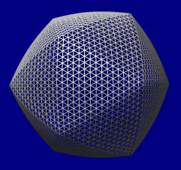### Icosahedron SurfaceIcosahedron Surface creates a surface representing a linear interpolation between an icosahedron and a sphere. The surface is for comparison to virus particles with icosahedral symmetry; it can be colored by density data for such structures with Surface Color. Icosahedron Surface information is saved in sessions. See also: shape icosahedron, hkcage, meshmol, Cage Builder

There are several ways to start Icosahedron Surface, a tool in the Higher-Order Structure category.

Clicking Show creates the surface according to the settings:

• Radius - radius of the sphere component and distance from the center to a vertex of the icosahedron component (the default is 100 display units, generally Å); the value can be changed by entering a different number or by moving the slider.
• Sphere factor - weight to use for the sphere component, where 0 (default) yields an icosahedron and 1 yields a sphere; the value can be changed by entering a different number or by moving the slider. Values outside the range 0-1 can be used, but negative values such that surface points pass through the origin will cause subsequent Sphere factor settings to produce the wrong surface.
• Orientation - orientation of the icosahedron
• xyz 2-fold axes (222) (default) - with two-fold symmetry axes of the icosahedron along the x, y and z axes. In the untransformed icosahedron, a vertically oriented edge points at the user. (Objects are untransformed when no rotations/translations have been performed, or when the view has been reset to the default.)
• xyz 2-fold axes, alt (222r) - as above, except rotated 90° about the z axis. In the untransformed icosahedron, a horizontally oriented edge points at the user.
• x 2-fold, z 3-fold (2n3) - with a two-fold symmetry axis of the icosahedron along the x axis and a three-fold symmetry axis along the z axis. The y axis does not coincide with a symmetry axis. A face of the untransformed icosahedron points at the user.
• x 2-fold, z 3-fold, alt (2n3r) - as above, except rotated 180° about the y axis.
• x 2-fold, z 5-fold (2n5) - with a two-fold symmetry axis of the icosahedron along the x axis and a five-fold symmetry axis along the z axis. The y axis does not coincide with a symmetry axis. A vertex of the untransformed icosahedron points at the user.
• x 2-fold, z 5-fold, alt (2n5r) - as above, except rotated 180° about the y axis.
• y 2-fold, z 5-fold (n25) - with a two-fold symmetry axis of the icosahedron along the y axis and a five-fold symmetry axis along the z axis. The x axis does not coincide with a symmetry axis. A vertex of the untransformed icosahedron points at the user.
• y 2-fold, z 5-fold, alt (n25r) - as above, except rotated 180° about the x axis.
• Subdivision factor - how finely to subdivide the surface. A factor of 1 (default) yields 20 triangles, the 20 faces of the icosahedron. Values represent the number of subdivisions within an edge of the icosahedron, and only non-negative powers of 2 are used (1, 2, 4, 8, ...). Other numbers can be entered, but will be rounded to a power of 2. The spacing is the length of a subdivision.
• Surface style - whether the surface should be shown as solid or mesh
• Color - a color well specifying the surface color
• Line width - pixel width of lines in the mesh style
Only one “icosahedral” surface can be present at a time. Changing any parameter automatically updates the surface. Remove closes this surface model, while Close dismisses the Icosahedron Surface dialog. Help opens this manual page in a browser window.

#### TECHNICAL NOTES

Rounded edges. Even when the Sphere factor is zero, the edges of the icosahedron may appear to be rounded because of the way the model is lit. This is especially noticable in the solid style. Each surface vertex has a normal vector that determines how light reflects off the surface. The vertices at the edges between faces of the icosahedron have a normal vector midway between the normals of the faces. This causes the edges to appear rounded instead of sharp. A higher Subdivision factor (e.g., 32 or 64) will make the edges look sharper.

Coloring. The Surface Color tool assigns each surface vertex a color; between vertices, the colors are interpolated. More detailed color variations will be apparent with a higher Subdivision factor.

UCSF Computer Graphics Laboratory / July 2014